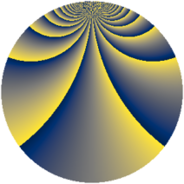# Properties

 Label 920.2.bqLevel $920$ Weight $2$ Character orbit 920.bq Rep. character $\chi_{920}(3,\cdot)$ Character field $\Q(\zeta_{44})$ Dimension $2800$ Newform subspaces $1$ Sturm bound $288$ Trace bound $0$

# Related objects

## Defining parameters

 Level: $$N$$ $$=$$ $$920 = 2^{3} \cdot 5 \cdot 23$$ Weight: $$k$$ $$=$$ $$2$$ Character orbit: $$[\chi]$$ $$=$$ 920.bq (of order $$44$$ and degree $$20$$) Character conductor: $$\operatorname{cond}(\chi)$$ $$=$$ $$920$$ Character field: $$\Q(\zeta_{44})$$ Newform subspaces: $$1$$ Sturm bound: $$288$$ Trace bound: $$0$$

## Dimensions

The following table gives the dimensions of various subspaces of $$M_{2}(920, [\chi])$$.

Total New Old
Modular forms 2960 2960 0
Cusp forms 2800 2800 0
Eisenstein series 160 160 0

## Trace form

 $$2800q - 18q^{2} - 36q^{3} - 28q^{6} - 18q^{8} + O(q^{10})$$ $$2800q - 18q^{2} - 36q^{3} - 28q^{6} - 18q^{8} - 18q^{10} - 72q^{11} - 30q^{12} - 44q^{16} - 36q^{17} - 14q^{18} - 22q^{20} - 52q^{22} - 36q^{25} - 36q^{26} - 60q^{27} + 30q^{28} - 38q^{30} + 2q^{32} - 12q^{33} - 36q^{35} - 36q^{36} - 26q^{38} + 2q^{40} - 72q^{41} - 242q^{42} - 36q^{43} - 4q^{46} - 26q^{48} + 38q^{50} - 104q^{51} + 142q^{52} - 84q^{56} - 60q^{57} - 42q^{58} + 38q^{60} - 42q^{62} - 36q^{65} + 12q^{66} - 36q^{67} - 108q^{68} - 24q^{70} + 4q^{72} - 36q^{73} - 36q^{75} - 76q^{76} + 30q^{78} - 160q^{80} + 112q^{81} - 10q^{82} + 44q^{83} - 52q^{86} + 14q^{88} - 420q^{90} - 160q^{91} - 42q^{92} - 124q^{96} - 36q^{97} - 26q^{98} + O(q^{100})$$

## Decomposition of $$S_{2}^{\mathrm{new}}(920, [\chi])$$ into newform subspaces

Label Dim. $$A$$ Field CM Traces $q$-expansion
$$a_2$$ $$a_3$$ $$a_5$$ $$a_7$$
920.2.bq.a $$2800$$ $$7.346$$ None $$-18$$ $$-36$$ $$0$$ $$0$$